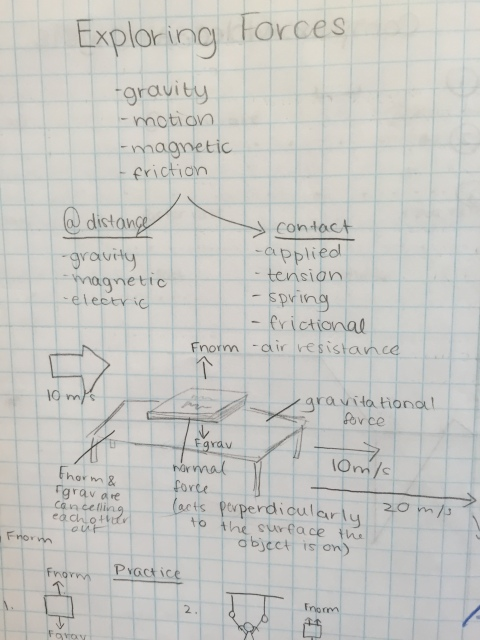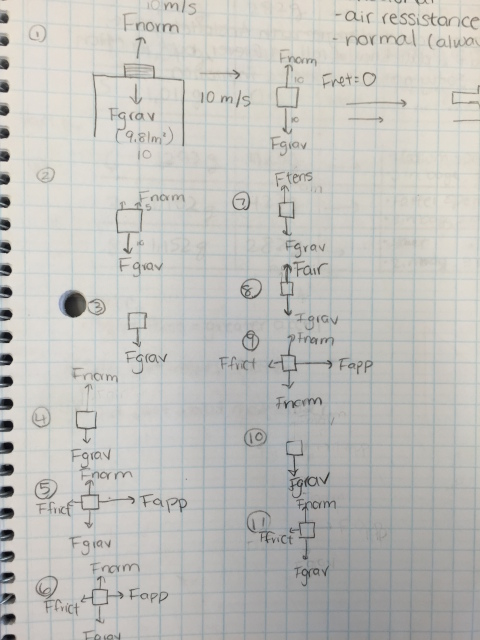# Exploring Forces (Part 1)

33 teachers like this lesson
Print Lesson

## Objective

SWBAT identify forces that act in the world and how they combine to create net force.

#### Big Idea

Students look at forces to understand why an object's motion either changes or does not change.

## Getting Started

In this lesson students investigate the relation between force, mass, and acceleration. Physicists use the formula F = ma (force = mass x acceleration) to represent these relations. This is Newton's second law of motion.

Our current way of looking at motion hasn't always been universally accepted.  Students will read about the history of Newton's laws, how they came to be recognized/explained, and why the second law of motion represents a major event in the history of science.

Students begin with a close look at forces to understand why an object's motion either changes or does not change. Using an understanding of these forces, they investigate the meaning of F = ma using a cart, different masses (rolls of pennies), an accelerometer, and videos.

Materials:

• carts
• tennis balls
• string
• accelerometers

## Engage

10 minutes

To begin the lesson ask students what they know about forces. Call on a few students to share their ideas. Move on to creating a list of the different types of forces they are familiar with.

From their list you can derive the following and organize it into two broad categories. Keep these posted in the class for the remainder of the lessons in this unit.

This list will be critical in future lessons so take the time to flush out these forces as best you can without explicitly telling them what they are. I do this by asking leading questions that hint at what the force might be. I think you'll find that most students can identify the majority of these forces even with a few hints.

Contact Forces                                          Action-at-a-Distance Forces

Frictional Force                                          Gravitational Force

Tension Force                                            Electrical Force

Normal Force                                             Magnetic Force

Air Resistance Force

Applied Force

Spring Force

Handout out the readings on forces and give students time to read and review. Note the diagrams on the Inventory of Forces reading. I like to have students copy the drawings to help put an image with the concept.## Explore Part 1

30 minutes

After reviewing the types of forces, ask your students to imagine pushing your cart on a level surface with one hand, and then stopping it with the other. Multiple forces would be involved in these actions.

What are they? What do you think is each force's direction and duration? When do you think each force comes into play? What effect do you think the forces are having on the cart?

Have students analyze the following force/motion scenarios with the cart, ball, and accelerometer. For each scenario they:

1. Act out the motion several times, observing carefully.
2. Sketch in their journal what happens, and why.
3. Describe each force as a vector, that is, identify the direction of the force and its relative magnitude (using arrows of different lengths).
4. Explain when and for how long each force is applied.
5. Describe the effect you think each force is having on the object.
6. Identify the net force on the object.
7. Describe the effect the net force is having on the object.

Remember that any time there is a change in motion (acceleration), there is a force causing it. The force causes an acceleration that is in the same direction as the force itself. Pay attention to whether there is more than one force involved and whether each force is an impulse force (a force that lasts for only a very short time, such as a push) or a force that acts for a longer time (such as friction).

In the video below I highlight the alignment of this lesson to the science practice of developing models which occurs in this lesson.

## Explore Part 2

20 minutes

In this section of the lesson students build off of their diagrams of the cart and ball and create force diagrams. Model for students how to construct the diagram. Start with a square then add vectors and labels for each force. Draw the vectors to show the approximate value of each force.

Give the students time to practice drawing then call for volunteers to come up and draw their diagrams on the board, or if you have individual white boards, have them all share using white boards.## Extend

20 minutes

To gain some historical perspective on our understanding of force and motion have your students read about the perspectives of Aristotle and Galileo. In this part of the lesson, students compare these two ideas and imagine how Aristotle and Galileo might respond to the model of the cart and ball and what they would say if they were a part of the lab group.